Moment of Inertia Calculator helps you easily find shapes of which you want to find the mass moment of inertia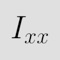Moment of Inertia Calculator

by Nolan Mathews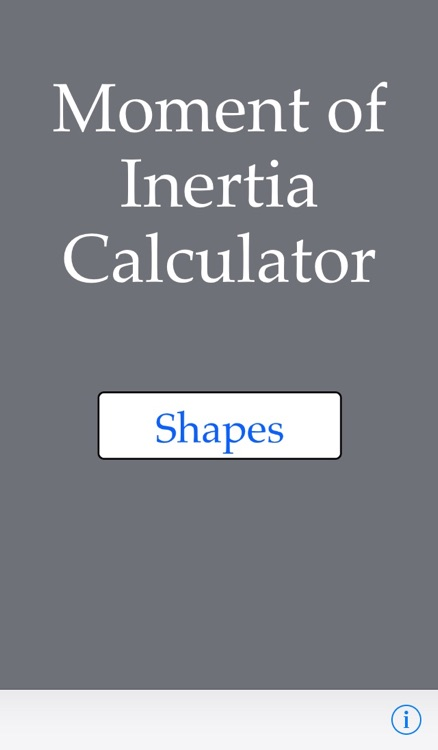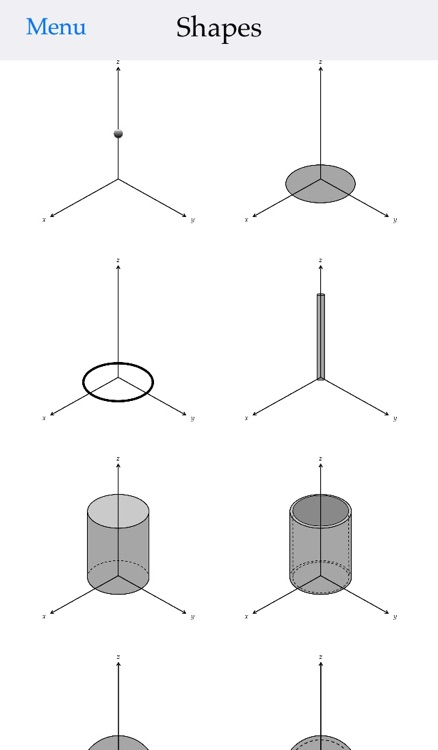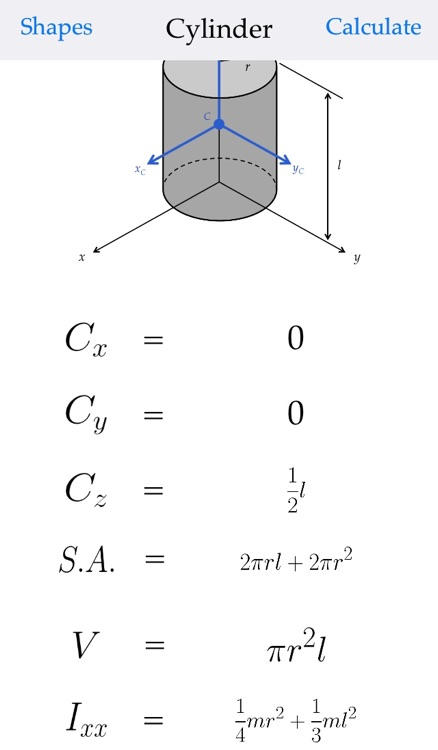Moment of Inertia Calculator helps you easily find shapes of which you want to find the mass moment of inertia. The app has two functions: "Equations" and "Calculate". The "Equations" function gives you the equations for the different mass moments of inertia, the centroid, the different radii of gyration, the centroid position, the volume, and the surface area of the desired shape. The "Calculate" function allows you to input the mass and dimensions of your specific shape and will calculate all of the terms given in the "Equations" function.App Details

Version
1.0
Rating
NA
Size
2Mb
Genre
Education Utilities
Last updated
September 24, 2014
Release date
September 24, 2014

App Screenshots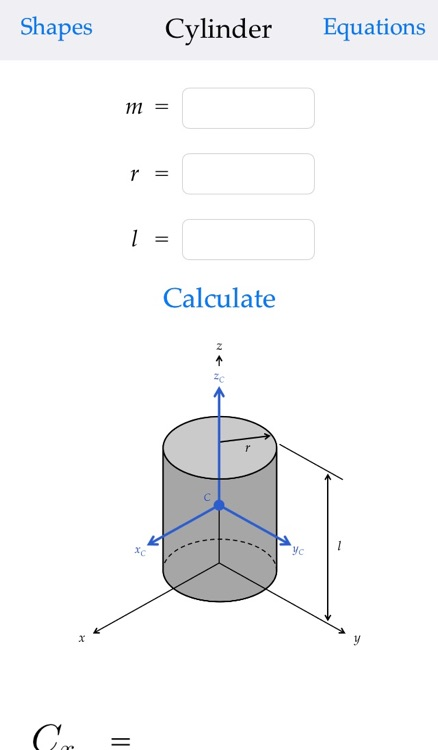App Store Description

Moment of Inertia Calculator helps you easily find shapes of which you want to find the mass moment of inertia. The app has two functions: "Equations" and "Calculate". The "Equations" function gives you the equations for the different mass moments of inertia, the centroid, the different radii of gyration, the centroid position, the volume, and the surface area of the desired shape. The "Calculate" function allows you to input the mass and dimensions of your specific shape and will calculate all of the terms given in the "Equations" function.

Disclaimer:
AppAdvice does not own this application and only provides images and links contained in the iTunes Search API, to help our users find the best apps to download. If you are the developer of this app and would like your information removed, please send a request to [email protected] and your information will be removed.